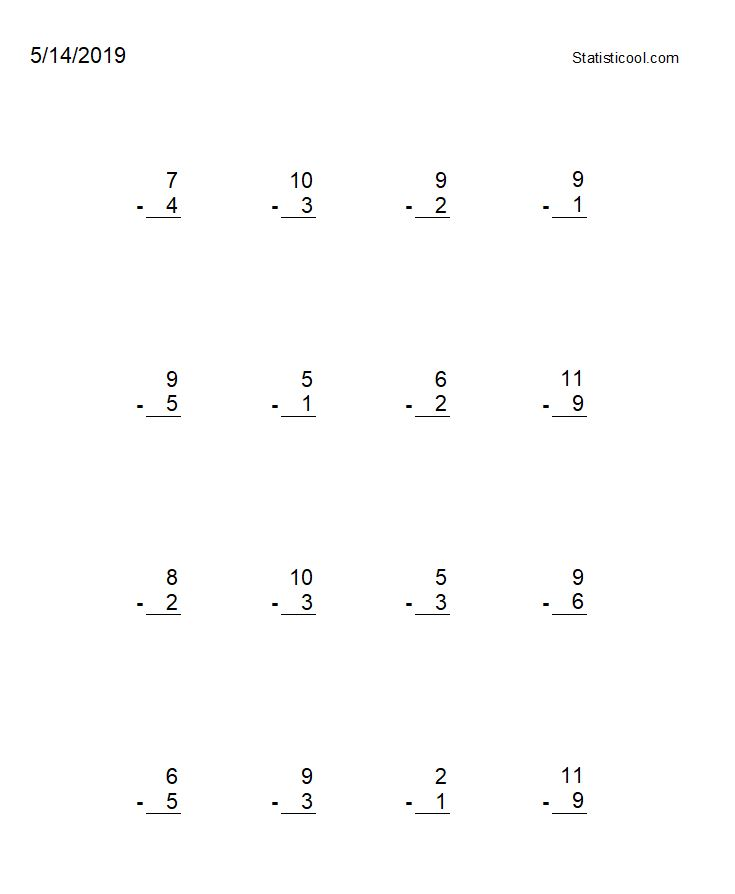If you find any of this useful, please consider donating via PayPal to help keep this site going.# Math Problem Generator

5/14/19

Here is a simple subtraction (and addition and multiplication) math problem generator for kids I created in Excel. I had it set up to create random subtraction problems using the numbers 0 through 10. If the top number is smaller than the bottom number in a subtraction problem, the result of course will be a negative number. However, smaller kids have not encountered that concept yet. Therefore I have logic in the spreadsheet to check if the top number is smaller than the bottom number, and if so, then flip the top and bottom. I also added today's date just for bookkeeping.

In the spreadsheet, if you print, the print area should be selected, and it will print out like the picture below. Re-entering the spreadsheet, or pressing F9, will refresh the random numbers to create new problems.Substraction shown here using numbers 1 through 11

As your child gets confident at problems using the numbers 0 through 10, just add larger numbers (11, 12, etc.) to the list. Or if small numbers (say 0, for example) are too easy, then remove them from the list.

Also, you can use this spreadsheet to create addition or multiplication problems, or a mix of them, by having "+" or "*" instead of, or in addition to, "-", in column B. The logic to randomly sample the operations is the same as the logic to randomly sample the numbers.

Some improvements that could be made to the spreadsheet are: one could edit and customize the logic to make it so 10 and 10 won't both be selected for a problem, and also make it so there are no duplicate problems on a page, or in a row.